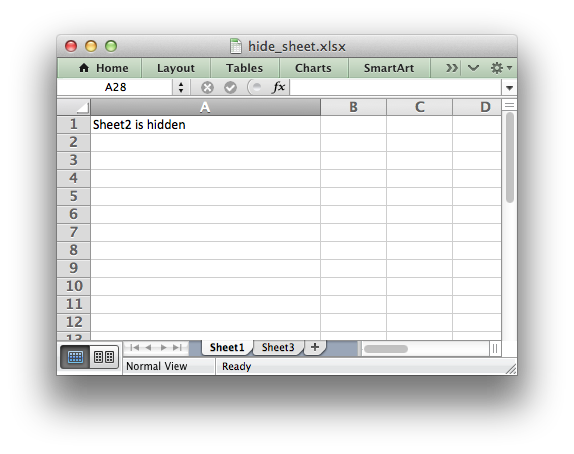libxlsxwriter
hide_sheet.c

Example of how to hide an Excel worksheet./*
* Example of how to hide a worksheet using libxlsxwriter.
*
* Copyright 2014-2021, John McNamara, jmcnamara@cpan.org
*
*/
#include "xlsxwriter.h"
int main() {
lxw_workbook *workbook = workbook_new("hide_sheet.xlsx");
lxw_worksheet *worksheet1 = workbook_add_worksheet(workbook, NULL);
lxw_worksheet *worksheet2 = workbook_add_worksheet(workbook, NULL);
lxw_worksheet *worksheet3 = workbook_add_worksheet(workbook, NULL);
/* Hide Sheet2. It won't be visible until it is unhidden in Excel. */
worksheet_hide(worksheet2);
worksheet_write_string(worksheet1, 0, 0, "Sheet2 is hidden", NULL);
worksheet_write_string(worksheet2, 0, 0, "Now it's my turn to find you!", NULL);
worksheet_write_string(worksheet3, 0, 0, "Sheet2 is hidden", NULL);
/* Make the first column wider to make the text clearer. */
worksheet_set_column(worksheet1, 0, 0, 30, NULL);
worksheet_set_column(worksheet2, 0, 0, 30, NULL);
worksheet_set_column(worksheet3, 0, 0, 30, NULL);
workbook_close(workbook);
return 0;
}
workbook_close
lxw_error workbook_close(lxw_workbook *workbook)
Close the Workbook object and write the XLSX file.
workbook_new
lxw_workbook * workbook_new(const char *filename)
Create a new workbook object.
lxw_worksheet
Struct to represent an Excel worksheet.
Definition: worksheet.h:2107
worksheet_hide
void worksheet_hide(lxw_worksheet *worksheet)
Hide the current worksheet.
lxw_workbook
Struct to represent an Excel workbook.
Definition: workbook.h:280
worksheet_write_string
lxw_error worksheet_write_string(lxw_worksheet *worksheet, lxw_row_t row, lxw_col_t col, const char *string, lxw_format *format)
Write a string to a worksheet cell.
worksheet_set_column
lxw_error worksheet_set_column(lxw_worksheet *worksheet, lxw_col_t first_col, lxw_col_t last_col, double width, lxw_format *format)
Set the properties for one or more columns of cells.